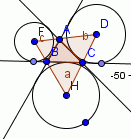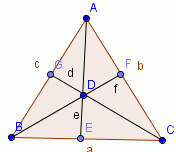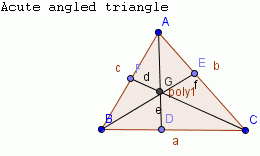Email us to get an instant 20% discount on highly effective K-12 Math & English kwizNET Programs!

#### Online Quiz (WorksheetABCD)

Questions Per Quiz = 2 4 6 8 10

### Grade 7 - Mathematics7.11 Excentres, Median, Centroid, Altitude and Orthocentre

Points to Remember:
1. An excircle or escribed circle of the triangle is a circle lying outside the triangle, tangent to one of its sides and tangent to the extensions of the other two. Every triangle has three distinct excircles, each tangent to one of the triangle's sides.

2. The centre of one of the excircles of a given triangle is known as the excentre. It is the point of intersection of one of the internal angle bisectors and two of the external angle bisectors of the triangle.

Example:Identify the excircles and the excentres in the above figure.

Solution:
In triangle ABC, the excircles are the 3 circles outside the triangle with excentres F, H and D respectively.

3. The medians of a triangle are the line segments joining the vertices of the triangle to the midpoints of the opposite sides.

4. The medians of a triangle are concurrent.

Example:Identify the median of the above triangle.

Solution:
In the triangle, 'AD' is the median.

5. The centroid of a triangle is the point of concurrence of its medians.

6. The centroid of a triangle divides the line segment joining any vertex to the midpoint of the opposite side in the ratio 2:1. In short centroid is a point of trisection of each median.

Example:Identify the centroid of the triangle given above.

Solution:
In the triangle, 'D' is the centroid.

7. Altitudes of a triangle are the perpendiculars drawn from the vertices of a triangle to the opposite sides.

8. The altitude of a triangle are concurrent.

Example:Identify the altitude in the given triangle

Solution:
In the triangle, 'AD' is the altitude.

9. The orthocenter is the point of concurrence of the altitudes of a triangle.

10. When the triangle is acute, then the orthocenter falls inside the triangle.

11. When the triangle is obtuse the orthocenter falls outside the triangle.

12. When the triangle is right angled, the orthocenter coincides with the vertex at which right angle is formed.

Example:.

Solution:
In the triangle the orthocentre is 'G'.

Hence we can conclude as follows.

 Median(The line segment joining the vertices of a triangle to the opposite sides.) Altitude(The perpendiculars drawn from the vertices of a triangle to the opposite sides.) Centroid The point of concurrence of the medians of a triangle. --------- Orthocentre ---------- The point of concurrence of the altitudes of a triangle.

Directions: Read the above review points carefully and answer the following questions:
1. Illustrate each of the above review points by drawing the excentres, the centroid and the orthocentre of different triangles.
2. Explain in your own words the different properties of excentres, centroid and circumcentre of a triangle, with examples.
3. Draw the centroid of a triangle with sides 5 cm, 9 cm and 7 cm.
4. Draw the orthocentre of triangle whose sides are given by 10 cm, 7 cm and 8 cm.

 Q 1: The ______ of a triangle is the point of concurrence of the altitudes of the triangle.orthocentreexcentrescentroid Q 2: Among the points the excentres, the circumcentre, the incentre, the orthocentre and the centroid, ________ may lie outside the triangle.the incentre and the centroidthe circumcentre and the orthocentrethe excentres Q 3: Among the points the excentres, the circumcentre, the incentre, the orthocentre and the centroid, ________ always lie outside the triangle.the excentresthe incentre and the centroidthe circumcentre and the orthocentre Q 4: Among the points the excentres, the circumcentre, the incentre, the orthocentre and the centroid. The points that always lie inside the triangle are _______.the incentre and the centroidthe excentresthe circumcentre and the orthocentre Q 5: The point of concurrence of the medians of a triangle is called the _______ of the triangle.excentrescentroidorthocentre Q 6: In an equilateral triangle the circumcentre, the orthocentre, the incentre and the centroid _________.coincidesare the excentresare midpoints Question 7: This question is available to subscribers only! Question 8: This question is available to subscribers only!

#### Subscription to kwizNET Learning System offers the following benefits:

• Unrestricted access to grade appropriate lessons, quizzes, & printable worksheets
• Instant scoring of online quizzes
• Progress tracking and award certificates to keep your student motivated
• Unlimited practice with auto-generated 'WIZ MATH' quizzes